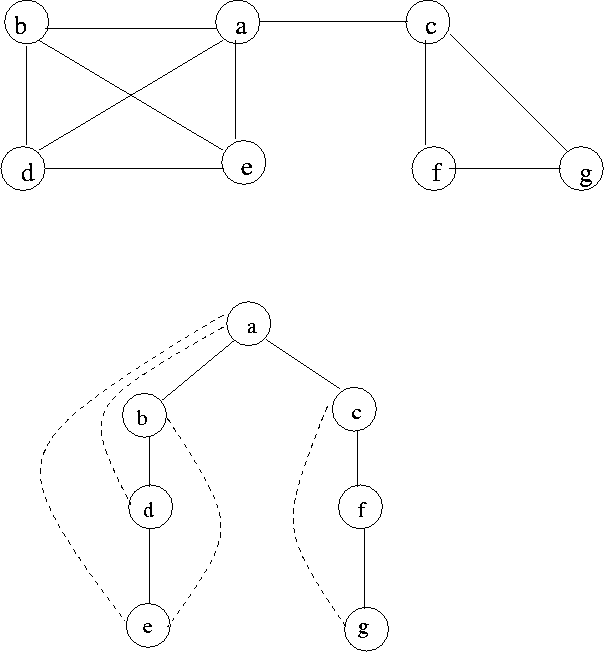Next:8.2.1 Breadth-first search of undirected graphUp:8. Undirected GraphsPrevious:8.1 Some Definitions

# 8.2 Depth First and Breadth First Search

See Figure 8.2 for an example. Assume the following adjacency lists.
 Vertex Adj. List a (b, c, d, e) b (a, d, e) c (a, f, g) d (a, b, e) e (a, b, d) f (c, g) g (c, f)• DFS of an undirected graph involves only two types of arcs.
• 1.
Tree arcs.
2.
Back arcs (there is no distinction between back arcs and forward arcs)
• Cross arcs also don't exist because given any two vertices, if there exists an arc between them, then one of them will be an ancestor and the other a descendant in the DFS. Thus all arcs that would have been cross arcs in the DFS of a digraph would become tree arcs in the case of an undirected graph.Next:8.2.1 Breadth-first search of undirected graphUp:8. Undirected GraphsPrevious:8.1 Some Definitions
eEL,CSA_Dept,IISc,Bangalore